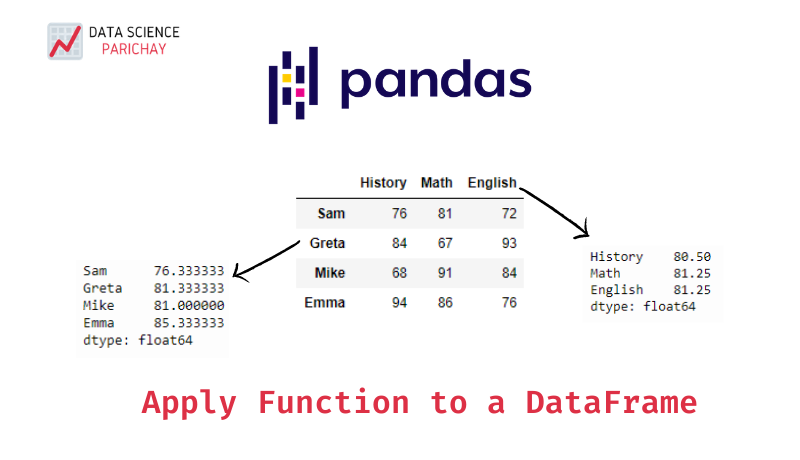# Apply a Function to a Pandas DataFrame

Pandas dataframes allow you the flexibility of applying a function along a particular axis of a dataframe. In this tutorial, we’ll look at how to apply a function to a pandas dataframe through some examples.

The pandas dataframe `apply()` function is used to apply a function along a particular axis of a dataframe. The following is the syntax:

``result = df.apply(func, axis=0)``

We pass the function to be applied and the axis along which to apply it as arguments. To apply the function to each column, pass `0` or `'index'` to the `axis` parameter which is `0` by default. And to apply the function to each row, pass `1` or `'columns'` to the `axis` parameter. The examples below illustrate the difference.

Let’s look at some of the use-cases of the `apply()` function through examples.

Let’s say you want to apply a function to each column of a dataframe, that is, along the index axis. For instance, you’re working with a dataframe having all numerical columns and you want to find the mean for each of those columns.

``````import pandas as pd

# create a sample dataframe
df = pd.DataFrame({
'History': [76, 84, 68, 94],
'Math': [81, 67, 91, 86],
'English': [72, 93, 84, 76]
})

# print the dataframe
print("The original dataframe:\n")
print(df)

# function to be applied
def get_mean(scores):
return sum(scores)/len(scores)

# get the mean score for each subject
result = df.apply(get_mean)
print("\nThe result of applying the function on the dataframe:\n")
print(result)``````

Output:

``````The original dataframe:

History  Math  English
0       76    81       72
1       84    67       93
2       68    91       84
3       94    86       76

The result of applying the function on the dataframe:

History    80.50
Math       81.25
English    81.25
dtype: float64``````

In the above example, the dataframe `df` contains scores of students in three subjects. Rows represent the students whereas columns represent the subjects. Here, the `apply()` function is used to get the average score for each of the subjects across all students. Note that, since the function `get_mean()` is applied to each column, we didn’t need to explicitly pass `0` to the `axis` parameter since it is its default value.

Now, let’s say you want to apply a function to each row of a dataframe, that is, along the columns axis. For instance, you’re working with a dataframe having all numerical rows and you want to find the mean for each of those rows.

📚 Data Science Programs By Skill Level

Introductory

Intermediate ⭐⭐⭐

🔎 Find Data Science Programs 👨‍💻 111,889 already enrolled

Disclaimer: Data Science Parichay is reader supported. When you purchase a course through a link on this site, we may earn a small commission at no additional cost to you. Earned commissions help support this website and its team of writers.

``````import pandas as pd

# create a sample dataframe
df = pd.DataFrame({
'History': [76, 84, 68, 94],
'Math': [81, 67, 91, 86],
'English': [72, 93, 84, 76]
}, index=['Sam', 'Greta', 'Mike', 'Emma'])

# print the dataframe
print("The original dataframe:\n")
print(df)

# function to be applied
def get_mean(scores):
return sum(scores)/len(scores)

# get the mean score for each student
result = df.apply(get_mean, axis=1)
print("\nThe result of applying the function on the dataframe:\n")
print(result)``````

Output:

``````The original dataframe:

History  Math  English
Sam         76    81       72
Greta       84    67       93
Mike        68    91       84
Emma        94    86       76

The result of applying the function on the dataframe:

Sam      76.333333
Greta    81.333333
Mike     81.000000
Emma     85.333333
dtype: float64``````

In the above example, the dataframe `df` contains scores of students in three subjects. Rows represent the students whereas columns represent the subjects. For more clarity, we give each row the name of its corresponding student. Here, the `apply()` function is used to get the average score for each student across all the three subjects. Note that, we had to pass `axis=1` since we wanted the `get_mean()` function to be applied to each row.

For more on the `apply()` function, refer to its official documentation.

With this, we come to the end of this tutorial. The code examples and results presented in this tutorial have been implemented in a Jupyter Notebook with a python (version 3.8.3) kernel having pandas version 1.0.5

•